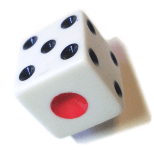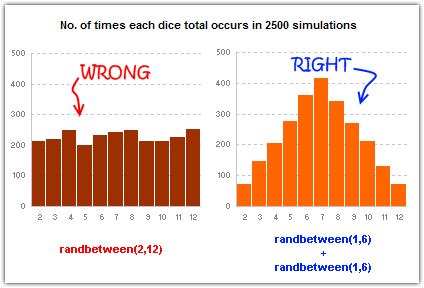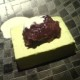# Simulating Dice throws – the correct way to do it in excel

ShareIf you ever had to simulate random outcomes in excel sheet, you might have already heard of about the spreadsheet function `rand()`, this little function generates a random fraction between 0 and 1 whenever you use it. So I usually write `=round(rand()*12,0)int(rand()*12)+1` if I need a random number between 0 to 12. Of course, if you have analysis tool pack installed like I do, then we can use `randbetween(0,12)` to do the same.

In order to simulate a dice throw, thus you can use `round(rand()*5,0)int(rand()*6)+1`.

So, what would you do if you need to simulate the face total when you throw 2 dice?

`round(rand()*10,0)int(rand()*11)+2` ?

Wrong

Why? Apparently a random number between 2 and 12 (1 is not possible as the minimum you can get when you throw two dice is 2) doesn’t simulate 2 dice throws properly.

The CORRECT way to do this is instead generate 2 individual random numbers and add them up, like:

`round(rand()*5,0)int(rand()*6) + 1 + round(rand()*5,0)int(rand()*6) + 1`

Here is why this is correct way to simulate dice throws using random number generator functions:

I have ran these 2 random functions each for 2500 times and plotted the distribution:As you can see, the left plot of `int(rand()*11)+2` tells that each of the 11 possibilities (2,3,4,5,6,7,8,9,10,11) are equally likely. But that is not what happens when you throw a dice, you see an awful lot more 5,6,7,8 than you see a perfect 12 or 2. And there is a reason for that, the distribution of 2 dice throws is actually a bell curve, and when you use `int(rand()*6) + int(rand()*6) + 2` the distribution is bell curvish.

Update: Thanks to Jon for pointing out that round() is not the choice if you want random integers, you should use int instead. See his explanation in the comments and the illustration here.

I have used this logic to simulate monopoly board game and prove that it is not really that random.

More on games: Bingo / Housie ticket generator excel sheet

### Get FREE Excel + Power BI Tips

Simple, fun and useful emails, once per week.

Learn & be awesome.

### Welcome to Chandoo.org

Thank you so much for visiting. My aim is to make you awesome in Excel & Power BI. I do this by sharing videos, tips, examples and downloads on this website. There are more than 1,000 pages with all things Excel, Power BI, Dashboards & VBA here. Go ahead and spend few minutes to be AWESOME.

Read my storyFREE Excel tips book

I will be presenting at two events in London in April 2020. Come & join me.

Chandoo is an awesome teacher
5/5

– Jason

Still on fence about Power BI? In this getting started guide, learn what is Power BI, how to get it and how to create your first report from scratch.

### 20 Responses to “Simulating Dice throws – the correct way to do it in excel”

1.alpha bravo says:

You have an interesting point, but the bell curve theory is nonsense. Certainly it is not what you would want, even if it were true.

2.Karl says:

Alpha Bravo - Although not a distribution curve in the strict sense, is does reflect the actual results of throwing two physical dice.

And reflects the following . .
There is 1 way of throwing a total of 2
There are 2 ways of throwing a total of 3
There are 3 ways of throwing a total of 4
There are 4 ways of throwing a total of 5
There are 5 ways of throwing a total of 6
There are 6 ways of throwing a total of 7
There are 5 ways of throwing a total of 8
There are 4 ways of throwing a total of 9
There are 3 ways of throwing a total of 10
There are 2 ways of throwing a total of 11
There is 1 way of throwing a total of 12

3.Chandoo says:

@alpha bravo ... welcome... 🙂

I am afraid the distribution shown in the right graph is what you get when you throw a pair of dice in real world. As Karl already explained, it is not random behavior you see when you try to combine 2 random events (individual dice throws), but more of order due to how things work.

@Karl, thanks 🙂

4.Jon Peltier says:

When simulating a coin toss, the ROUND function you used is appropriate. However, your die simulation formula should use INT instead of ROUND:

=INT(RAND()*6)+1

Otherwise, the rounding causes half of each number's predictions to be applied to the next higher number. Also, you'd get a count for 7, which isn't possible in a die.

To illustrate, I set up 1200 trials of each formula in a worksheet and counted the results. The image here shows the table and a histogram of results:

http://peltiertech.com/WordPress/wp-content/img200808/RandonDieTrials.png

5.Chandoo says:

@Jon: thanks for pointing this out. You are absolutely right. INT() is what I should I have used instead of ROUND() as it reduces the possibility of having either 1 or 6 by almost half that of having other numbers.

this is such a good thing to learn, helps me a lot in my future simulations.

Btw, the actual graphs I have shown were plotted based on randbetween() and not from rand()*6, so they still hold good.

Updating the post to include your comments as it helps everyone to know this.

6.Jon Peltier says:

By the way, the distribution is not a Gaussian distribution, as Karl points out. However, when you add the simulations of many dice together (i.e., ten throws), the overall results will approximate a Gaussian distribution. If my feeble memory serves me, this is the Central Limit Theorem.

7.Chandoo says:

@Jon, that is right, you have to nearly throw infinite number of dice and add their face counts to get a perfect bell curve or Gaussian distribution, but as the central limit theorem suggests, our curve should roughly look like a bell curve... 🙂

8. [...] posts on games & excel that you may enjoy: Simulating Dice throws in Excel Generate and Print Bingo / Housie tickets using this excel Understanding Monopoly Board [...]

9. [...] Correct way to simulate dice throws in excel [...]

10. [...] Simulate dice throws in excel [...]

11.YourFifthGradeMathsTeacher says:

I'm afraid to say that this is a badly stated and ambiguous post, which is likely to cause errors and misunderstanding.
Aside from the initial use of round() instead of int(),.. (you've since corrected), you made several crucial mistakes by not accurately and unambiguously stating the details.

Firstly, you said:
"this little function generates a random fraction between 0 and 1"
Correctly stated this should be:
"this little function generates a random fraction F where 0 <= F < 1".

Secondly, I guess because you were a little fuzzy about the exact range of values returned by rand(), you have then been just as ambiguous in stating:
"I usually write int(rand()*12)+1 if I need a random number between 0 to 12".
(that implies 13 integers, not 12)

Your formula, does not return 13 integers between 0 to 12.
It returns 12 integers between 1 and 12 (inclusive).
-- As rand() returns a random fraction F where 0 <= F < 1, you can obviously can only get integers between 1 and 12 (inclusive) from your formula as stated above, but clearly not zero.

"I usually write int(rand()*12) if I need a random number between 0 to 11 (inclusive)",
or:
"I usually write int(rand()*12)+1 if I need a random number between 1 to 12 (inclusive)"
then you would have been correct.

12.Justin says:

Idk if I'm on the right forum for this or how soon one can reply, but I'm working on a test using Excel and I have a table set up to get all my answers from BUT I need to generate 10,000 answers from this one table. Every time, I try to do this I get 10,000 duplicate answers. I know there has to be some simple command I have left out or not used at all, any help would be extremely helpful! (And I already have the dice figured out lol)

Roll 4Dice with 20Sides (4D20) if the total < 20 add the sum of a rerolled 2D20. What is the average total over 10,000 turns? (Short and sweet)

Like I said when I try to simulate 10,000turns I just get "67" 10,000times -_- help please! 😀

13.Hui... says:

@Justin

This is a good example to use for basic simulation

have a look at the file I have posted at:
https://rapidshare.com/files/1257689536/4_Dice.xlsx

It uses a variable size dice which you set
Has 4 Dice
Throws them 10,000 times
If Total per roll < 20 uses the sum of 2 extra dice Adds up the scores Averages the results You can read more about how it was constructed by reading this post: http://chandoo.org/wp/2010/05/06/data-tables-monte-carlo-simulations-in-excel-a-comprehensive-guide/

14.SpreadSheetNinja says:

Oh derp, i fell for this trap too, thinking i was makeing a good dice roll simulation.. instead of just got an average of everything 😛

Noteably This dice trow simulate page is kinda important, as most roleplay dice games were hard.. i mean, a crit failure or crit hit (rolling double 1's or double 6's) in a a game for example dungeons and dragons, if you dont do the roll each induvidual dice, then theres a higher chance of scoreing a crit hit or a crit failure on attacking..

15.Freswinn says:

I've been working on this for awhile. So here's a few issues I've come across and solved.

#1. round() does work, but you add 0.5 as the constant, not 1.

trunc() and int() give you the same distributions as round() when you use the constant 1, so among the three functions they are all equally fair as long as you remember what you're doing when you use one rather than the other. I've proven it with a rough mathematical proof -- I say rough only because I'm not a proper mathematician.

In short, depending on the function (s is the number of sides, and R stands in for RAND() ):

round(f), where f = sR + 0.5
trunc(f), where f = sR + 1
int(f), where f = sR + 1

will all give you the same distribution, meaning that between the three functions they are fair and none favors something more than the others. However...

#2. None of the above gets you around the uneven distribution of possible outcomes of primes not found in the factorization of the base being used (base-10, since we're using decimal; and the prime factorization of 10 is 2 and 5).

With a 10-sided die, where your equation would be
=ROUND(6*RAND()+0.5)
Your distribution of possible values is even across all ten possibilities.
However, if you use the most basic die, a 6-sided die, the distributions favor some rolls over others. Let's assume your random number can only generate down to the thousandths (0.000 ? R ? 0.999). The distribution of possible outcomes of your function are:
1: 167
2: 167
3: 166
4: 167
5: 167
6: 166

So 4 and 6 are always under-represented in the distribution by 1 less than their compatriots. This is true no matter how many decimals you allow, though the distribution gets closer and closer to equal the further towards infinite decimal places you go.
This carries over to all die whose numbers of sides do not factor down to a prime factorization of some exponential values of 2 and 5.

So, then, how can we fix this one, tiny issue in a practical manner that doesn't make our heads hurt or put unnecessary strain on the computer?

16.Freswinn says:

Real quick addendum to the above:
Obviously when I put the equation after the example of the 10-sided die, I meant to put a 10*RAND() instead of a 6*RAND(). Oops!

Also, where I have 0.000 ? R ? 0.999, the ?'s are supposed to be less-than-or-equal-to signs but the comments didn't like that. Oh well.

17.Andrew says:

How do you keep adding up the total? I would like to have a cell which keeps adding up the total sum of the two dices, even after a new number is generated in the cells when you refresh or generate new numbers.

18.kk says:

So, how do you simulate rolling 12 dice? Do you write int(rand()*6) 12 times?

Is there a simpler way of simulating n dice in Excel?

19.Mohammed Ali says:

I've run this code in VBA

Sub generate()
Application.ScreenUpdating = False
Application.Calculation = False
Dim app, i As Long
Set app = Application.WorksheetFunction

For i = 3 To 10002
Cells(i, 3).Value = i - 2
Cells(i, 4).Value = app.RandBetween(2, 12)
Cells(i, 5).Value = app.RandBetween(1, 6) + app.RandBetween(1, 6)
Next
Application.ScreenUpdating = True
Application.Calculation = True
End Sub

But I get the same distribution for both columns 4 and 5
Why ?

•Hui... says:

@Mohammed

I would expect to get the same distribution as you have effectively used the same function

### Get FREE Excel & Power-BI Newsletter

One email per week with Excel and Power BI goodness. Join 100,000+ others and get it free.